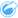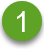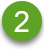# Calculate Density

Calculate Density uses input point features to calculate a density map within an area of interest. Calculate Density uses a Kernel density calculation to create a density surface from your point features.

## Examples

Bird counts can be used to calculate species densities. The densities can then be compared to land-cover data to determine which habitats each species prefers.

## Use the Calculate Density capability

Calculate Density can be run on maps with point layers.

Use the following steps to run the Calculate Density analysis capability:

1. Si es necesario, haga clic en la tarjeta de mapa para activarla. Una tarjeta está activa cuando aparecen la barra de herramientas y el botón Acción.
2. Click the Action button and choose Calculate Density.
3. For Choose a point layer, select the layer for which you want to calculate density.
4. Expand Additional options and enter values for the Weight, Search radius (bandwidth), and Cell size parameters, if necessary. See Usage notes for more information.
5. Click Run.

## Usage notes

The Choose a point layer parameter is used to select a dataset to calculate densities. Only point features are available in the drop-down menu.

Expand Additional options to reveal the Weight, Search radius (bandwidth), and Cell size parameters. The following table summarizes these parameters, including their default values:

ParameterDescriptionDefault value

Weight

A field denoting the value of each feature. For example, if you have a dataset for retail locations that includes a field for revenue, you could use the revenue field as the weight to create a density surface based on sales amount rather than locations.

All features will be weighted as 1 (in other words, the density surface will be based solely on the location of the features).

A distance (in miles, feet, kilometers, or meters) that is used to find input features within the same neighborhood as the focal feature.

An appropriate search distance will be calculated for the input dataset using Silverman's Rule-of-thumb formula.

Cell size

The size of the output features that will create the density surface.

An appropriate cell size will be calculated for the input dataset based on the extent of the dataset and number of features.

The result dataset will be styled by Counts and Amounts (Color) using a default equal interval classification with 10 classes.

## Limitations

Densities can only be calculated for point features.

## How Calculate Density works

Calculate Density uses a Kernel density calculation to create a density surface. The following sections explain the Kernel density calculation, as well as the default calculations for Search radius (bandwidth) and Cell size.

### Kernel density

Kernel density calculates the density of features within a circular neighborhood surrounding each output cell using a Gaussian function. Conceptually, a smooth curved surface is fitted over each point. The surface value is highest at the location of the point and diminishes with increasing distance from the point, reaching zero when the distance from the point equals the search distance.One of the points in the dataset.A distance equal to the search radius (bandwidth).

Each surface also includes volume. The volume for the surface is equal to the Weight parameter for each feature, or 1 if no value is specified. The weight determines how many times a point is counted in the density formula.

The density for each cell is calculated by adding the values of all the kernel surfaces where they overlay the output cell center. The kernel function is based on the Quartic kernel function described in Silverman (1986, p. 76, Equation 4.5).

The following formula is used to calculate density at (x,y) location:

where:

• ρ = The density at (x,y) location.
• r = The search radius (bandwidth).
• i = 1, ..., n input points. Only points within the search radius of the (x,y) location are included.
• Wi = The weight of point i. If a weight field is not specified, the weight will be 1 for all points.
• di = The distance between point i and the (x,y) location. The distance must be less than the search radius.

For more information on the formula, see Formulas for calculating Kernel Density.

The default search radius applies an algorithm to your data based on both the extent of your data and the density of the points. The Search radius (bandwidth) field appears blank because the default radius is not calculated until the analysis begins. When you leave the Search radius (bandwidth) field blank, the default radius is applied. For more information, see Default search radius (bandwidth) algorithm.

If you prefer to specify your own search radius, consider that the larger the search radius, the more generalized the pattern. A smaller search radius shows more local variation but may miss the broader picture.

### Cell size

If a cell size is not provided, the cell size will be calculated based on formulas described in Hengl (2006). The formulas are dataset dependent and are chosen based on the number of features and the extent or scale of the input dataset to optimize both performance and output resolution.

### Distance calculations

Calculate Density can be run using either a projected coordinate system (projected data) or a geographic coordinate system (nonprojected data). When projected data is used, a Euclidean distance (a straight-line distance measured on a flat surface) is calculated. When nonprojected data is used, a geodesic distance (a line drawn on a sphere representing the curvature of the earth) is calculated. The geodesic distance calculation is based on Haversine's formula.

## References

Silverman, B. W. (1986). Density Estimation for Statistics and Data Analysis. Chapman and Hall.

Hengl, T. (2006). Finding the right pixel size. Computers & Geosciences, 32(9), 1283-1298. https://doi.org/10.1016/j.cageo.2005.11.008